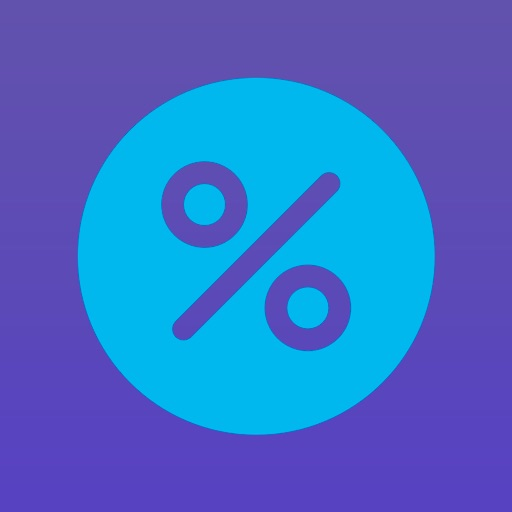## PercentiCal is an easy to use and intuitive percentage calculator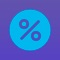# PercentiCal - Add & Deduct %

by Filipp Zimin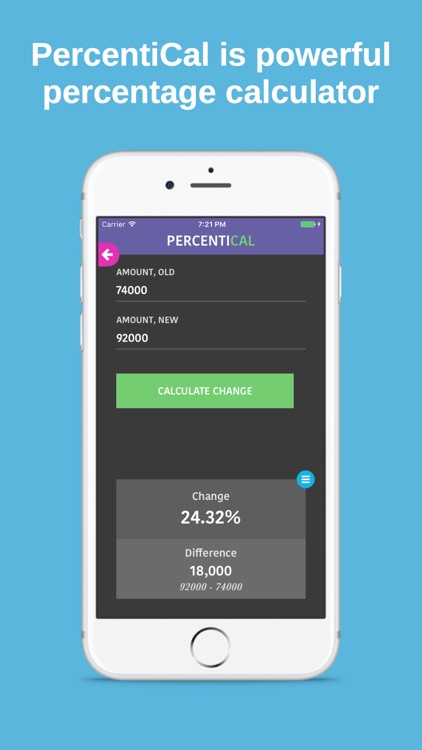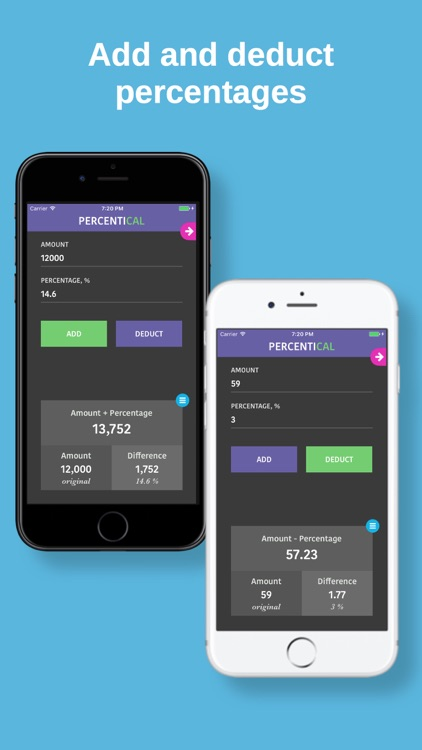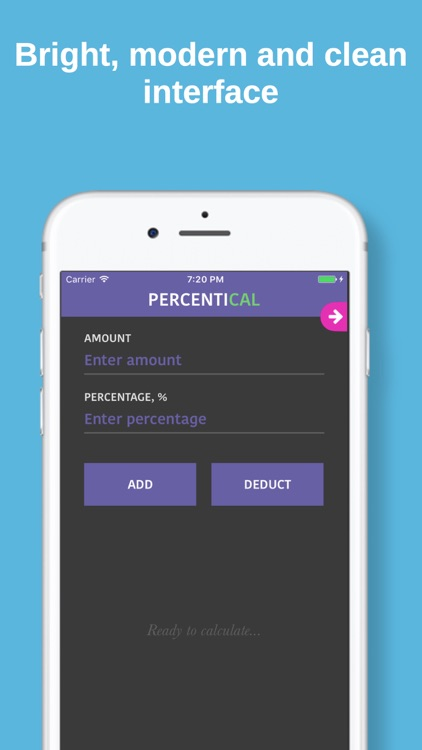PercentiCal is an easy to use and intuitive percentage calculator. It’s simple and clean design lets you add and subtract percentages (for example discounts). PercentiCal can also calculate a percentage change between two numbers.### App Details

Version
1.2
Rating
NA
Size
15Mb
Genre
Last updated
September 23, 2017
Release date
June 26, 2017

### App Screenshots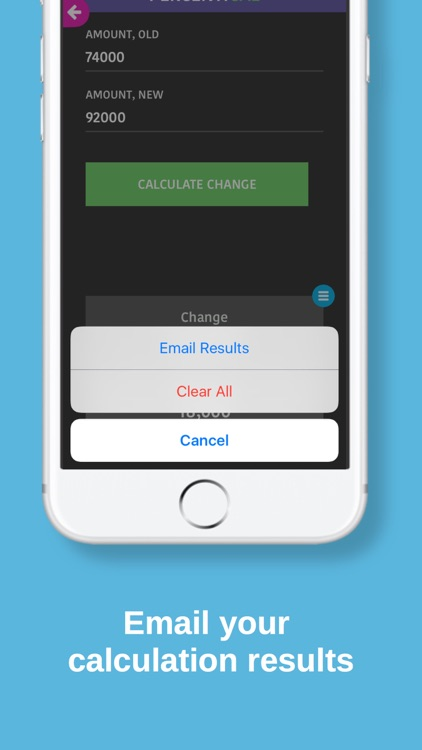### App Store Description

PercentiCal is an easy to use and intuitive percentage calculator. It’s simple and clean design lets you add and subtract percentages (for example discounts). PercentiCal can also calculate a percentage change between two numbers.

Features: